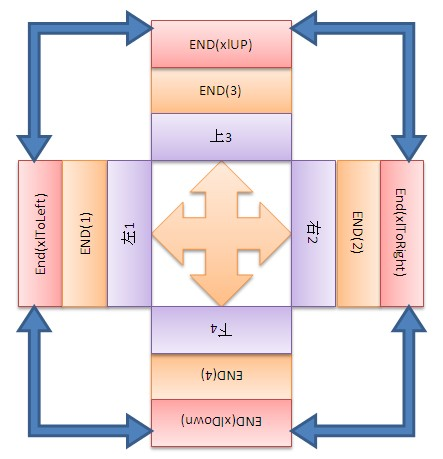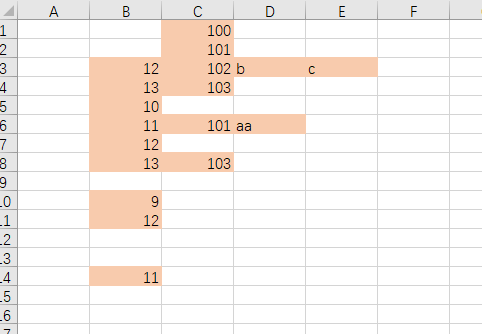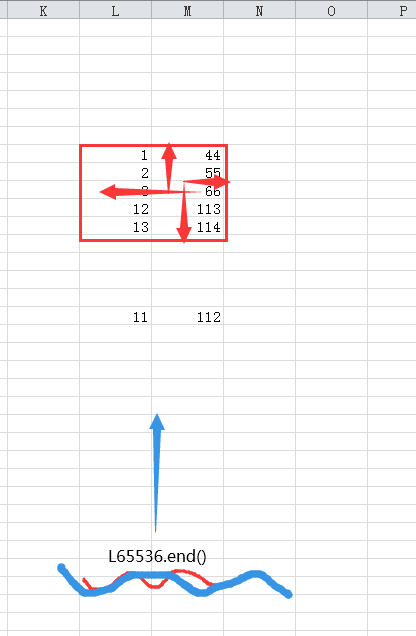# 1 Range().End() 或 cells().End()  属性

### 1.1 单元格的end属性

• 两种写法都可以
• range().end()
• cells().end()

### 1.2 返回的内容

• 返回一个 Range 对象
• 该对象代表包含源区域的区域尾端的单元格。
• 等同于按键 (End+向上键、End+向下键、End+向左键、End+向右键)
• 或等同于CTRL+上下左右

# 2 使用详解

### 2.1 详细语法

• 表达式 一个代表 Range 对象的变量。（用数值1,2,3,4 代表也是可以的）
• 向左 xlToLeft -------1，实际使用时，是以当前range()为基点，先左移一格，然后去找
• 向右 xlToRight -----2，实际使用时，是以当前range()为基点，先右移一格，然后去找
• 向上 xlUp -----------3，实际使用时，是以当前range()为基点，先上移一格，然后去找
• 向下 xlDown -------4，实际使用时，是以当前range()为基点，先下移一格，然后去找• 理解先偏移1格再找区域的意思
• 理解从“源区域” 作为起始点，去找这个 源区域相连的使用区域的边界
• 如果源区域 本身为空，会找一个相连的整块空区域sub test1()

'确定的区域，测试单元格1
Debug.Print Range("b5").End(xlUp).Row
Debug.Print Range("b5").End(xlDown).Row
Debug.Print Range("b5").End(xlToLeft).Column
Debug.Print Range("b5").End(xlToRight).Column
Debug.Print

'确定的区域，测试单元格1
Debug.Print Range("b3").End(xlUp).Row
Debug.Print Range("b3").End(xlDown).Row
Debug.Print Range("b3").End(xlToLeft).Column
Debug.Print Range("b3").End(xlToRight).Column
Debug.Print

end sub

### 2.2 测试比较

• 查固定range()的范围
• 查固定range()，但range()内全部为空
• 查不确定的区域的范围
Sub test_end1()

Debug.Print Range("b:b").Rows.Count
'Debug.Print Range("b:b").maxrowCount
Debug.Print Range("3:3").Columns.Count
Debug.Print

'确定的区域，这样查也是有意义的
'返回一个 Range 对象，它表示包含源范围的区域末尾的单元格
Debug.Print Range("b1:b5").End(xlUp).Row
Debug.Print Range("b1:b5").End(xlDown).Row
Debug.Print Range("b1:b5").End(xlToLeft).Column
Debug.Print Range("b1:b5").End(xlToRight).Column
Debug.Print

'空区域
Debug.Print Range("d1:d5").End(xlUp).Row
Debug.Print Range("d1:d5").End(xlDown).Row
Debug.Print Range("d1:d5").End(xlToLeft).Column
Debug.Print Range("d1:d5").End(xlToRight).Column
Debug.Print

'查不确定的区域
Debug.Print Range("b:b").End(xlUp).Row
Debug.Print Range("b:b").End(xlDown).Row
Debug.Print Range("b:b").End(xlToLeft).Column
Debug.Print Range("b:b").End(xlToRight).Column
Debug.Print

'查不确定的区域，用处比较大
'比如查B列的上下限界。因为中间可能有空格隔断，所以得这么查
Debug.Print "查B列的上下限界,从列的开始往下查，从列的末尾往上查"
Debug.Print Range("b1").End(xlDown).Row
Debug.Print Range("b65536").End(xlUp).Row

'比如查3行的左右限界。因为中间可能有空格隔断，所以得这么查
Debug.Print "查第3行的左右限界，从行的开始往右查，从行的结尾（尽量大的数）往左边查"
Debug.Print Range("a3").End(xlToRight).Column
Debug.Print Cells(3, 9999).End(xlToLeft).Column

End Sub

### 2.3 查不确定区域的范围的正确用法（不是从内部查边界，而是从4个外界去逼近边界）

'查不确定的区域，用处比较大
'比如查B列的上下限界。因为中间可能有空格隔断，所以得这么查
Debug.Print "查B列的上下限界,从列的开始往下查，从列的末尾往上查"
Debug.Print Range("b1").End(xlDown).Row
Debug.Print Range("b65536").End(xlUp).Row

'比如查3行的左右限界。因为中间可能有空格隔断，所以得这么查
Debug.Print "查第3行的左右限界，从行的开始往右查，从行的结尾（尽量大的数）往左边查"
Debug.Print Range("a3").End(xlToRight).Column
Debug.Print Cells(3, 9999).End(xlToLeft).Column

# 3 总结

## 3.1 利用end() 查内部的边界

• 查一个连续区域内的边界，在区域内，用这些即可
• end(xlup)
• end(xldown)
• end(xltoleft)
• end(xltorigjt)
• 查一个连续区域内部

## 3.2 利用end() 查外部的边界（比如查某列最后一个非空单元格）

• 从外部逼近单元格，需要从  大的行列反过来逼近
• Range("a65536").End(xlUp)适合查整个sheet的某一列的最后一个有值得单元格。# 4   新版EXCEL里，一般用rows.count 代替 a65536

• Range("a65536").End(xlUp).Row
• cells(rows.count,1).end(xlup).row

01-215605
04-13803
12-024136
08-202202
12-314678
01-251万+
12-272047
05-06
06-18

### “相关推荐”对你有帮助么？

•非常没帮助
•没帮助
•一般
•有帮助
•非常有帮助被折叠的  条评论 为什么被折叠?到【灌水乐园】发言¥2 ¥4 ¥6 ¥10 ¥20余额支付 (余额：-- )扫码支付获取中扫码支付点击重新获取扫码支付1.余额是钱包充值的虚拟货币，按照1:1的比例进行支付金额的抵扣。
2.余额无法直接购买下载，可以购买VIP、C币套餐、付费专栏及课程。余额充值